Next: Born Approximation Up: Scattering Theory Previous: Introduction

# Fundamental Equations

Consider time-independent scattering theory, for which the Hamiltonian of the system is written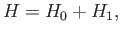(10.1)

where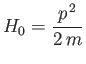(10.2)

is the Hamiltonian of a free particle of mass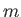, and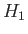represents the non-time-varying source of the scattering. Let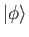be an energy eigenket of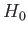,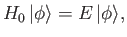(10.3)

whose wavefunction is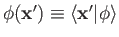. This wavefunction is assumed to be a plane wave. Schrödinger's equation for the scattering problem is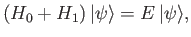(10.4)

where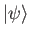is an energy eigenstate of the total Hamiltonian whose wavefunction is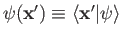. In general, bothand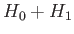have continuous energy spectra: that is, their energy eigenstates are unbound. We require a solution of Equation (10.4) that satisfies the boundary condition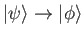as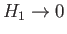. Here,is a solution of the free-particle Schrödinger equation, (10.3), that corresponds to the same energy eigenvalue as.

Adopting the Schrödinger representation (see Section 2.4), we can write the scattering equation, (10.4), in the form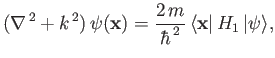(10.5)

where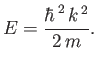(10.6)

(See Exercise 1.) Equation (10.5) is known as the Helmholtz equation, and can be inverted using standard Green's function techniques . Thus,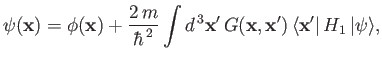(10.7)

where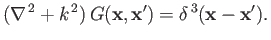(10.8)

(See Exercise 2.) Here,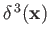is a three-dimensional Dirac delta function. Note that the solution (10.7) satisfies the previously mentioned constraintas. As is well known, the Green's function for the Helmholtz equation is given by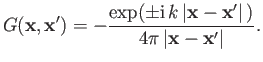(10.9)

(See Exercise 3.) Thus, Equation (10.7) becomes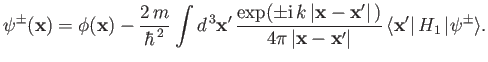(10.10)

Let us suppose that the scattering Hamiltonian,, is a function only of the position operators. This implies that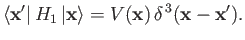(10.11)

We can write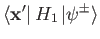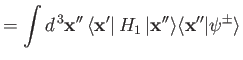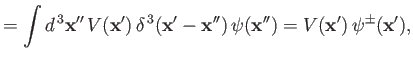(10.12)

where use has been made of the standard completeness relation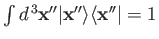. (See Section 1.15.) Thus, the integral equation (10.10) simplifies to give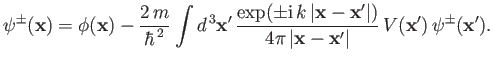(10.13)

Suppose that the initial state,, possesses a plane-wave wavefunction with wavevector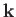(i.e., it corresponds to a stream of particles of definite momentum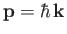). The ket corresponding to this state is denoted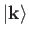. Thus,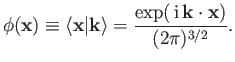(10.14)

The preceding wavefunction is conveniently normalized such that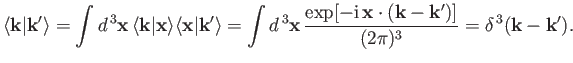(10.15)

(See Section 2.6 and Exercise 4.)

Suppose that the scattering potential,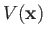, is non-zero only in some relatively localized region centered on the origin (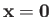). Let us calculate the total wavefunction,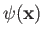, far from the scattering region. In other words, let us adopt the ordering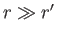, where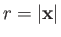and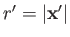. It is easily demonstrated that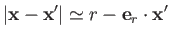(10.16)

to first order in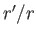, where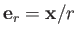is a unit vector that is directed from the scattering region to the observation point. Let us define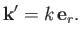(10.17)

Clearly,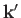is the wavevector for particles that possess the same energy as the incoming particles (i.e.,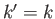), but propagate from the scattering region to the observation point. Note that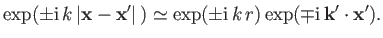(10.18)

In the large-limit, Equations (10.13) and (10.14) reduce to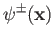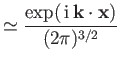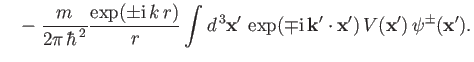(10.19)

The first term on the right-hand side of the previous equation is the incident wave. The second term represents a spherical wave centered on the scattering region. The plus sign (on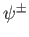) corresponds to a wave propagating away from the scattering region, whereas the minus sign corresponds to a wave propagating toward the scattering region. (See Exercise 5.) It is obvious that the former represents the physical solution. Thus, the wavefunction far from the scattering region can be written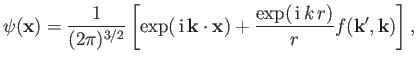(10.20)

where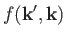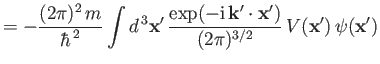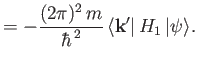(10.21)

[See Equations (10.11) and (10.14).]

Let us define the differential scattering cross-section,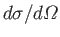, as the number of particles per unit time scattered into an element of solid angle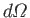, divided by the incident particle flux. Recall, from Chapter 3, that the probability current (which is proportional to the particle flux) associated with a wavefunction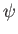is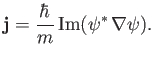(10.22)

Thus, the particle flux associated with the incident wavefunction,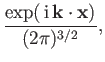(10.23)

is proportional to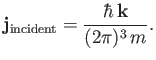(10.24)

Likewise, the particle flux associated with the scattered wavefunction,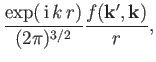(10.25)

is proportional to(10.26)

Now, by definition,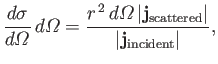(10.27)

giving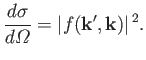(10.28)

Thus,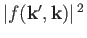is the differential cross-section for particles with incident momentum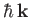to be scattered into states whose momentum vectors are directed in a range of solid anglesabout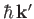. Note that the scattered particles possess the same energy as the incoming particles (i.e.,). This is always the case for scattering Hamiltonians of the form specified in Equation (10.11).Next: Born Approximation Up: Scattering Theory Previous: Introduction
Richard Fitzpatrick 2016-01-22